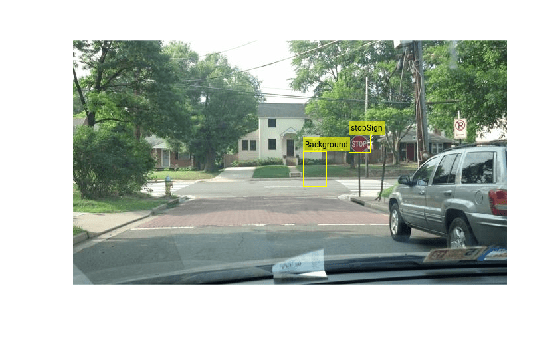# classifyRegions

Classify objects in image regions using R-CNN object detector

## Syntax

``````[labels,scores] = classifyRegions(detector,I,rois)``````
``````[labels,scores,allScores] = classifyRegions(detector,I,rois)``````
``[___] = classifyRegions(___Name,Value)``

## Description

example

``````[labels,scores] = classifyRegions(detector,I,rois)``` classifies objects within the regions of interest of image `I`, using an R-CNN (regions with convolutional neural networks) object detector. For each region, `classifyRegions` returns the class label with the corresponding highest classification score.When using this function, use of a CUDA® enabled NVIDIA® GPU is highly recommended. The GPU reduces computation time significantly. Usage of the GPU requires Parallel Computing Toolbox™. For information about the supported compute capabilities, see GPU Support by Release (Parallel Computing Toolbox).```
``````[labels,scores,allScores] = classifyRegions(detector,I,rois)``` also returns all the classification scores of each region. The scores are returned in an M-by-N matrix of M regions and N class labels.```
````[___] = classifyRegions(___Name,Value)` specifies options using one or more `Name,Value` pair arguments. For example, `classifyRegions(detector,I,rois,'ExecutionEnvironment','cpu')` classifies objects within image regions using only the CPU hardware.```

## Examples

collapse all

`load('rcnnStopSigns.mat','rcnn')`

`img = imread('stopSignTest.jpg');`

Specify multiple regions to classify within the test image.

```rois = [416 143 33 27 347 168 36 54]; ```

Classify regions.

```[labels,scores] = classifyRegions(rcnn,img,rois); detectedImg = insertObjectAnnotation(img,'rectangle',rois,cellstr(labels)); figure imshow(detectedImg)```## Input Arguments

collapse all

R-CNN object detector, specified as an `rcnnObjectDetector` object. To create this object, call the `trainRCNNObjectDetector` function with training data as input.

Input image, specified as a real, nonsparse, grayscale or RGB image.

Data Types: `uint8` | `uint16` | `int16` | `double` | `single` | `logical`

Regions of interest within the image, specified as an M-by-4 matrix defining M rectangular regions. Each row contains a four-element vector of the form [x y width height]. This vector specifies the upper left corner and size of a region in pixels.

### Name-Value Arguments

Specify optional pairs of arguments as `Name1=Value1,...,NameN=ValueN`, where `Name` is the argument name and `Value` is the corresponding value. Name-value arguments must appear after other arguments, but the order of the pairs does not matter.

Before R2021a, use commas to separate each name and value, and enclose `Name` in quotes.

Example: `'MiniBatchSize',64`

Example: `'ExecutionEnvironment','cpu'`

Size of smaller batches for R-CNN data processing, specified as the comma-separated pair consisting of `'MiniBatchSize'` and an integer. Larger batch sizes lead to faster processing but take up more memory.

Hardware resource used to classify image regions, specified as the comma-separated pair consisting of `'ExecutionEnvironment'` and `'auto'`, `'gpu'`, or `'cpu'`.

• `'auto'` — Use a GPU if it is available. Otherwise, use the CPU.

• `'gpu'` — Use the GPU. To use a GPU, you must have Parallel Computing Toolbox and a CUDA enabled NVIDIA GPU. If a suitable GPU is not available, the function returns an error. For information about the supported compute capabilities, see GPU Support by Release (Parallel Computing Toolbox).

• `'cpu'` — Use the CPU.

## Output Arguments

collapse all

Classification labels of regions, returned as an M-by-1 categorical array. M is the number of regions of interest in `rois`. Each class name in `labels` corresponds to a classification score in `scores` and a region of interest in `rois`. `classifyRegions` obtains the class names from the input `detector`.

Highest classification score per region, returned as an M-by-1 vector of values in the range [0, 1]. M is the number of regions of interest in `rois`. Each classification score in `scores` corresponds to a class name in `labels` and a region of interest in `rois`. A higher score indicates higher confidence in the classification.

All classification scores per region, returned as an M-by-N matrix of values in the range [0, 1]. M is the number of regions in `rois`. N is the number of class names stored in the input `detector`. Each row of classification scores in `allscores` corresponds to a region of interest in `rois`. A higher score indicates higher confidence in the classification.

## Version History

Introduced in R2016b